[BACK]
 Computers, Materials & ContinuaDOI:10.32604/cmc.2022.030067Article

Weather Forecasting Prediction Using Ensemble Machine Learning for Big Data Applications

1Department of Computer Sciences, College of Computer and Information Sciences, Princess Nourah Bint Abdulrahman University, Riyadh, 11671, Saudi Arabia
2Department of Information Systems, College of Computer and Information Sciences, Princess Nourah Bint Abdulrahman University, Riyadh, 11671, Saudi Arabia
3Department of Computer Sciences, College of Computing and Information System, Umm Al-Qura University, Saudi  Arabia
4Department of Computer Science, College of Science & Art at Mahayil, King Khalid University, Saudi Arabia
5Faculty of Science, Mathematics and Computer Science Department, Menoufia University, Egypt
6Department of Computer and Self Development, Preparatory Year Deanship, Prince Sattam bin Abdulaziz University, AlKharj, Saudi Arabia
7Research Centre, Future University in Egypt, New Cairo, 11845, Egypt
*Corresponding Author: Anwer Mustafa Hilal. Email: a.hilal@psau.edu.sa
Received: 17 March 2022; Accepted: 19 April 2022

Abstract: The agricultural sector’s day-to-day operations, such as irrigation and sowing, are impacted by the weather. Therefore, weather constitutes a key role in all regular human activities. Weather forecasting must be accurate and precise to plan our activities and safeguard ourselves as well as our property from disasters. Rainfall, wind speed, humidity, wind direction, cloud, temperature, and other weather forecasting variables are used in this work for weather prediction. Many research works have been conducted on weather forecasting. The drawbacks of existing approaches are that they are less effective, inaccurate, and time-consuming. To overcome these issues, this paper proposes an enhanced and reliable weather forecasting technique. As well as developing weather forecasting in remote areas. Weather data analysis and machine learning techniques, such as Gradient Boosting Decision Tree, Random Forest, Naive Bayes Bernoulli, and KNN Algorithm are deployed to anticipate weather conditions. A comparative analysis of result outcome said in determining the number of ensemble methods that may be utilized to improve the accuracy of prediction in weather forecasting. The aim of this study is to demonstrate its ability to predict weather forecasts as soon as possible. Experimental evaluation shows our ensemble technique achieves 95% prediction accuracy. Also, for 1000 nodes it is less than 10 s for prediction, and for 5000 nodes it takes less than 40 s for prediction.

Keywords: Weather; forecasting; KNN; random forest; gradient boosting decision tree; naive bayes bernoulli

1  Introduction

In Meteorological data, a huge volume of data is collected by various sensors for the prediction of weather forecasting. Gathering such big data is a vital process that aids people’s day-to-day activities. Prediction of weather conditions is essential for human beings to plan mitigation measures for them from harmful weather conditions. It is also useful in many areas, including decision-making, agriculture, tourism, and business [1,2]. Big Data contains tremendous weather information in an unstructured, semi-organized format. Handling this unstructured data is a difficult task to process and store. Therefore, a machine learning technique is implemented. The current prediction of weather forecasting models depends on complicated physical models which need high-performance computer systems with many HPC nodes for the implementation of the prediction systems. Despite the employment of high-performance computers and high-speed communication lines, such systems yield erroneous forecasts or an incomplete understanding of atmospheric processes. Furthermore, running such a sophisticated model takes more time . To address the drawbacks of existing models, the proposed method employs a variety of algorithms and ensembles them using a maximum voting mechanism. It is reliable, accurate, and has a minimum prediction time and error rate.

Machine learning methods are also used in weather forecasting classification. There is machine learning techniques exist that have the ability to predict the weather conditions such as rainfall, wind speed, wind direction, and temperature. Random forest, KNN, Gradient boost algorithm, decision tree, and other machine learning techniques are combined to build a machine learning algorithm, which is referred to as an ensemble-based technique. The advantage of using the ensemble-based technique is to increase the prediction accuracy and produces better results.

The main contributions of our proposed ensemble-based weather forecasting (EBWF)model are:

•   An ensemble-based prediction technique is proposed for enhanced weather prediction performance.

•   Gradient boosting technique is developed for identifying relevant features for accurate weather prediction. This feature selection approach requires minimum time complexity.

This article is organized as follows: Section 2 provides a review of several research literature, Section 3 describes the prediction of weather forecasting using an ensemble-based approach of max voting, Section 4 discusses the results, and Section 5 concludes the research with future directions.

2  Literature Review

The use of classical and deep learning algorithms to forecast weather temperature has been widely investigated in the literature. The majority of the work relies on supervised learning techniques. Historical data of meteorological factors such as past temperature and wind speed and direction are used to forecast the weather. The support vector machine (SVM) and artificial neural network (ANN) are the most common models used in weather forecasting so far. The capacity of CNN to deal with non-linear and high-dimensional data is well-known. SVM is noted for its accuracy and resilience. Based on previous studies, prior temperature, relative humidity, solar radiation, rain, and wind speed observations are the top attributes for forecasting temperature among the available meteorological attributes. Among the most commonly utilized performance measurements are Mean Squared Error (MSE) and Mean Absolute Error (MAE). Notice that some models are dedicated to predicting hourly temperatures whereas others predict longer-term temperatures such as 24 h ahead .

The authors in  released a publicly available weather dataset and conducted simple models as a baseline enabling others to run new models and compare their findings with the existing models to further research in weather prediction up to several days ahead. The dataset includes weather data between 1979 and 2018. The dataset includes features such as temperature, humidity, wind, cloud cover, precipitation, and solar radiation. In addition to constant variables such as the soil type, longitude, and latitude. Regression, deep learning, and physical prediction models are among the baseline models presented in the study. The outcomes of the experiments are reported using several performance metrics. When dealing with weather forecasts, the authors recommend utilizing a successive period of time for testing and validating the models rather than using random samples of data. Therefore, the authors used the year 2016 for validating the performance of their models, the years 2017 and 2018 for testing, and all years from 1979to 31 December 2016 for training the models. The authors mentioned some challenges and future directions for research and that included, selecting the best combination of features, applying different machine learning techniques, dealing with big data, and having larger weather datasets to improve weather forecasting.

The WRF model (Weather Research and Forecasting) is a complex numeric weather prediction model. Short-term and long-term prediction models were implemented using the deep learning models named long short-term memory (LSTM) and temporal convolutional network (TCN) . The results are compared with the WRF model. LSTM and TCN models were first implemented for short-term weather forecasts. They were then fine-tuned for long-term predictions. The study proposes two different forms of models. The first model employs a single network with 10 inputs and outputs. That is, it predicts future values of all-weather predictors based on their historical values. The second model, on the other hand, implies 10 separate networks, each with ten inputs and only one output. This indicates that each network receives historical values for all-weather attributes, but only forecasts the future value of one attribute at a time. The training dataset includes the duration from January to May 2018. The testing data covers the period of June 2018 while the validation set includes the period of July 2018. The results show that proposed deep learning-based models outperform the WRF model with the advantage of having a lightweight model compared to WRF. They also show that having a network for each prediction produces better results than having one network that produces all the forecasts.

The proposed model in  combines numerical weather prediction (NWP) with historical data to forecast the weather. The study uses deep learning called the deep uncertainty quantification model (UQM) and optimizes its performance by using a loss function that is based on the negative log-likelihood error (NLE). The data is pre-processed. First, records with entirely missing data are deleted otherwise linear interpolation is used to impute missing data. Continuous features are normalized using min-max normalization into [0, 1]. Categorical data are encoded by embedding. Three weather features are used in the proposed model which are the temperature and relative humidity at 2 meters and wind at 10 meters. Tab. 1 describes that survey on the prediction of Weather forecasting.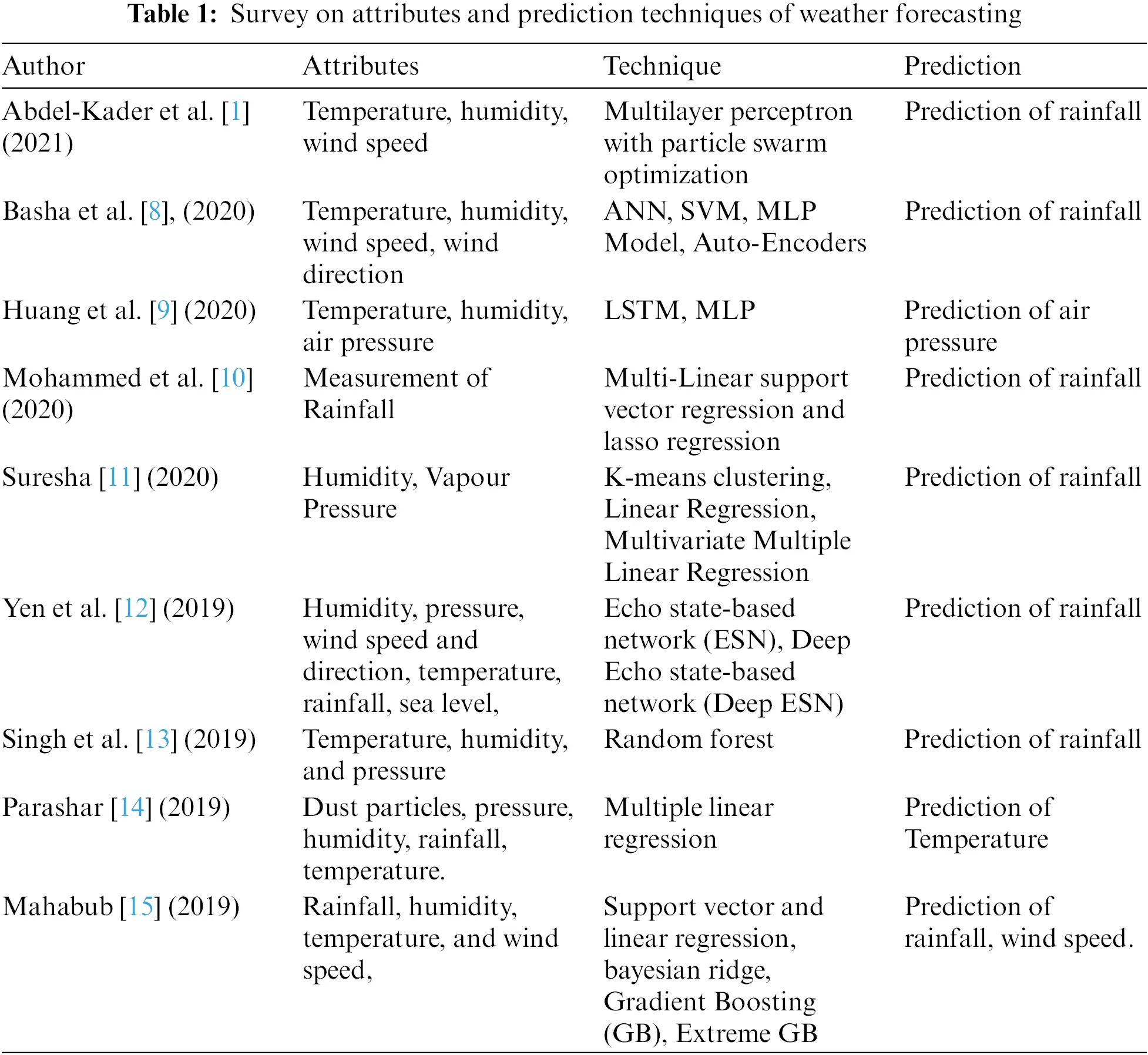3  Proposed Methodology

The proposed framework has been developed with all components including weather data collection, pre-processing, feature selection, ensemble-based evolutionary model building, and evaluation of the prediction results. Fig. 1 explains the weather forecasting proposed framework. Ensemble learning is an advanced machine learning technique that combines the results of several machine learning algorithms. This will lead to a better prediction of weather forecasting compared to single machine learning algorithms.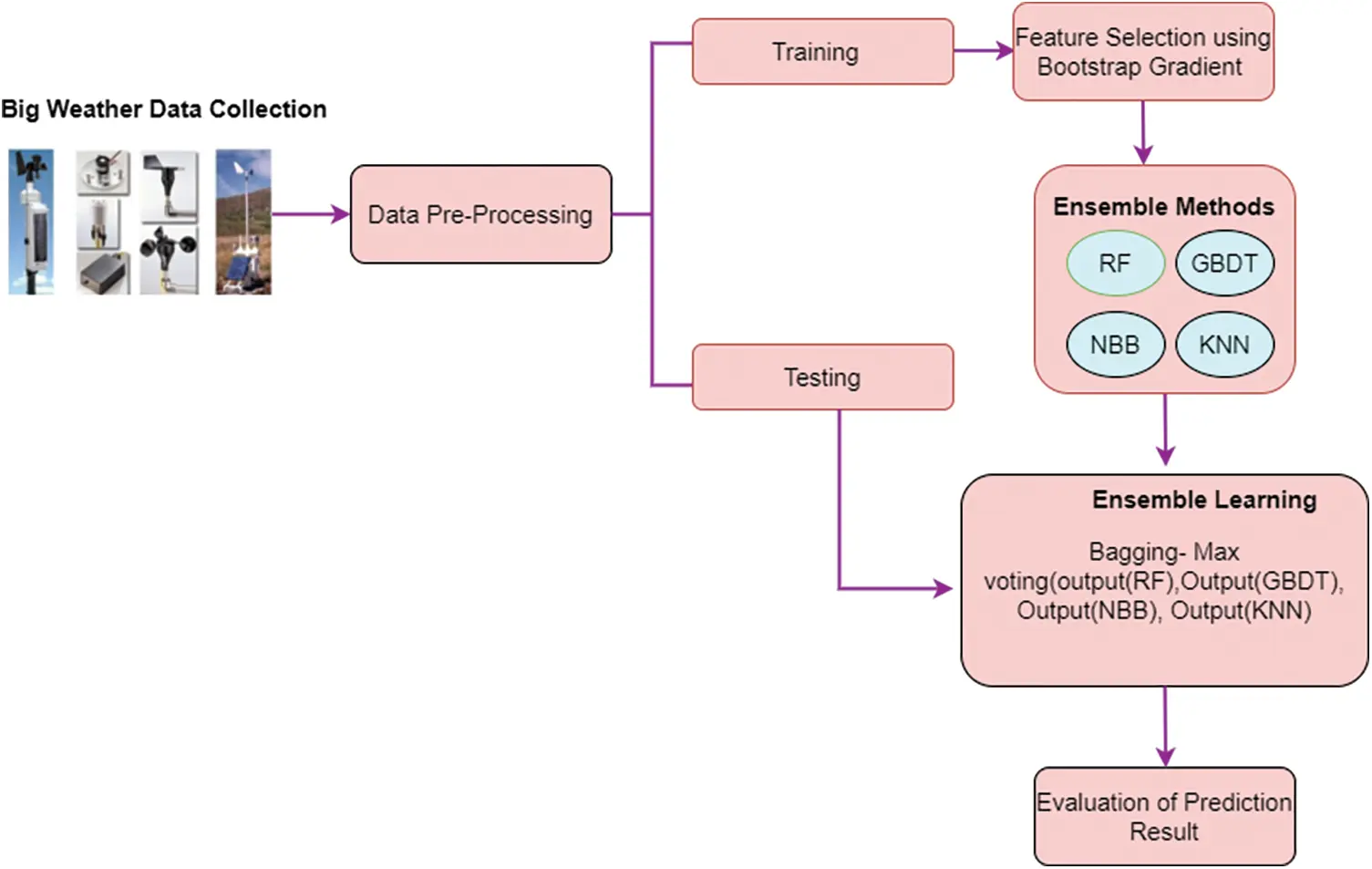Figure 1: Weather forecasting framework

The weather data set is divided into training data and testing data. The data is pre-processed to fill missing values and perform data normalization. The features are identified using gradient boosting which uses a gradient descent algorithm. The selected features are then classified using ensemble machine learning (ML) algorithms such as random forest, gradient boosting decision tree, Naive Bayes Bernoulli, and k-nearest neighbor (KNN) Algorithm to predict weather conditions. The prediction results of the above algorithms are ensemble using the bagging method which is called max-voting for the final prediction result.

3.1 Data Collection

The data is collected from various airport weather stations in India . This dataset includes various attributes of air temperature, atmospheric pressure, humidity, the direction of the wind, and other variables. The sample attribute of weather data is given in Tab. 2. The experiments are implemented using Python version 3.7.3 with the Tensorflow version. The dataset includes contains 2006–2018 with 9 Features. Our model forecasts the weather 3 h ahead of time. The training dataset includes all features from the year 2006-to 2016. The testing dataset includes the years 2017 and 2018 with selected features in the dataset. Fig. 2 shows the feature representation of the weather data set in every 3 h which contains 27 features.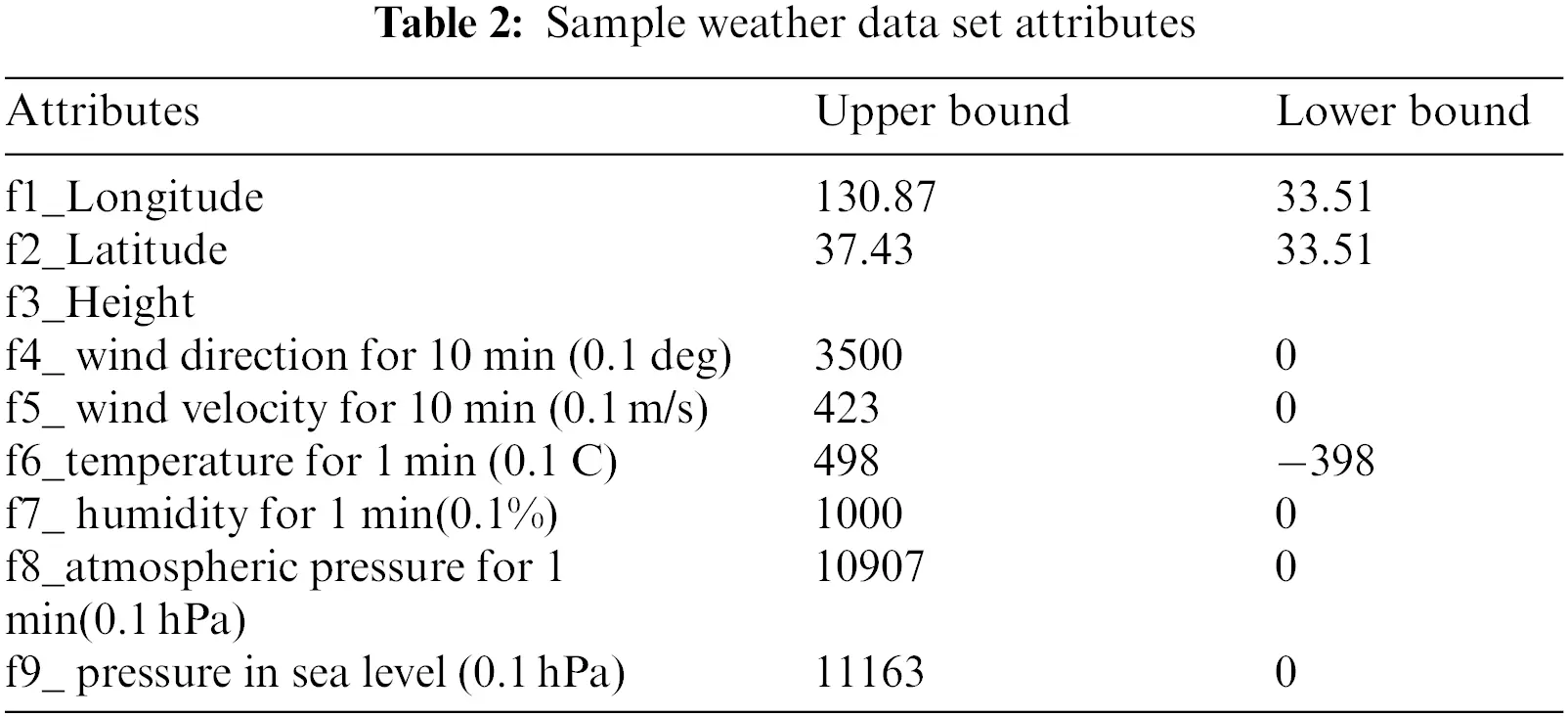Figure 2: Feature representation for every 3 h

3.2 Pre-Processing

The collected weather dataset contains invalid or empty values. Therefore, the pre-processing stage is necessary. This stage includes data cleaning, data normalization, and one-hot encoding. Fig. 3 shows the phases of pre-processing.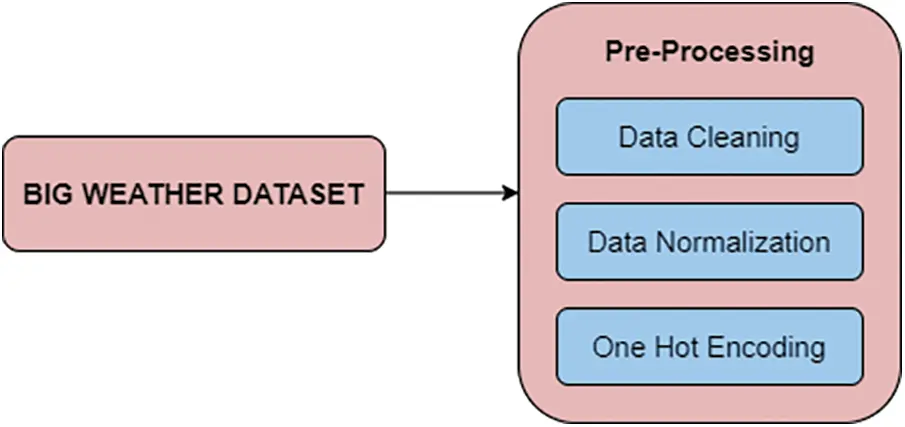Figure 3: Phases in pre-processing

In data cleaning, raw weather data contains noise, inconsistent values, and missing values which affect the accuracy of the weather forecasting. In order to improve the quality and performance of the result. The null values are identified and eliminated from the data set. In this work, Min-Max normalization is used. The weather dataset is scaled into a range normalization] or [−1, 1]. This Min-Max normalization meta attributed the input of the attribute values o dnorm and the range of val is between [min,max] by using the following formula:

dnorm=(maxmin)(dlow)highlow(1)

Here max is the maximum value of the selected attribute and min is the minimum value of the selected attribute. dnorm is the newly selected feature after applying the normalization. The benefit of normalization is to have data consistency.

One hot encoding converts the categorical feature of wind direction and its condition with dummy variables. This conversion is needed in the training and testing data set for keeping the same number of attribute features. That is by giving 1 for the presence of attribute and 0 for the absence of the attribute in the dataset.

3.3 Feature Selection

There are various feature selection algorithms like information gain, correlation, gain ratio, and so on. Such algorithms are used to identify important features from the whole feature set. This proposed concept uses an ensemble learning method called gradient boosting for relevant features selection. The bootstrap samples are independent and distributed evenly with the minimum correlation between the sample weather data. The gradient boosting technique uses gradient descent steps to reduce the loss while including input data into the ensemble model. Gradient descent is similar to the random forest but different in the way that samples are chosen without replacement.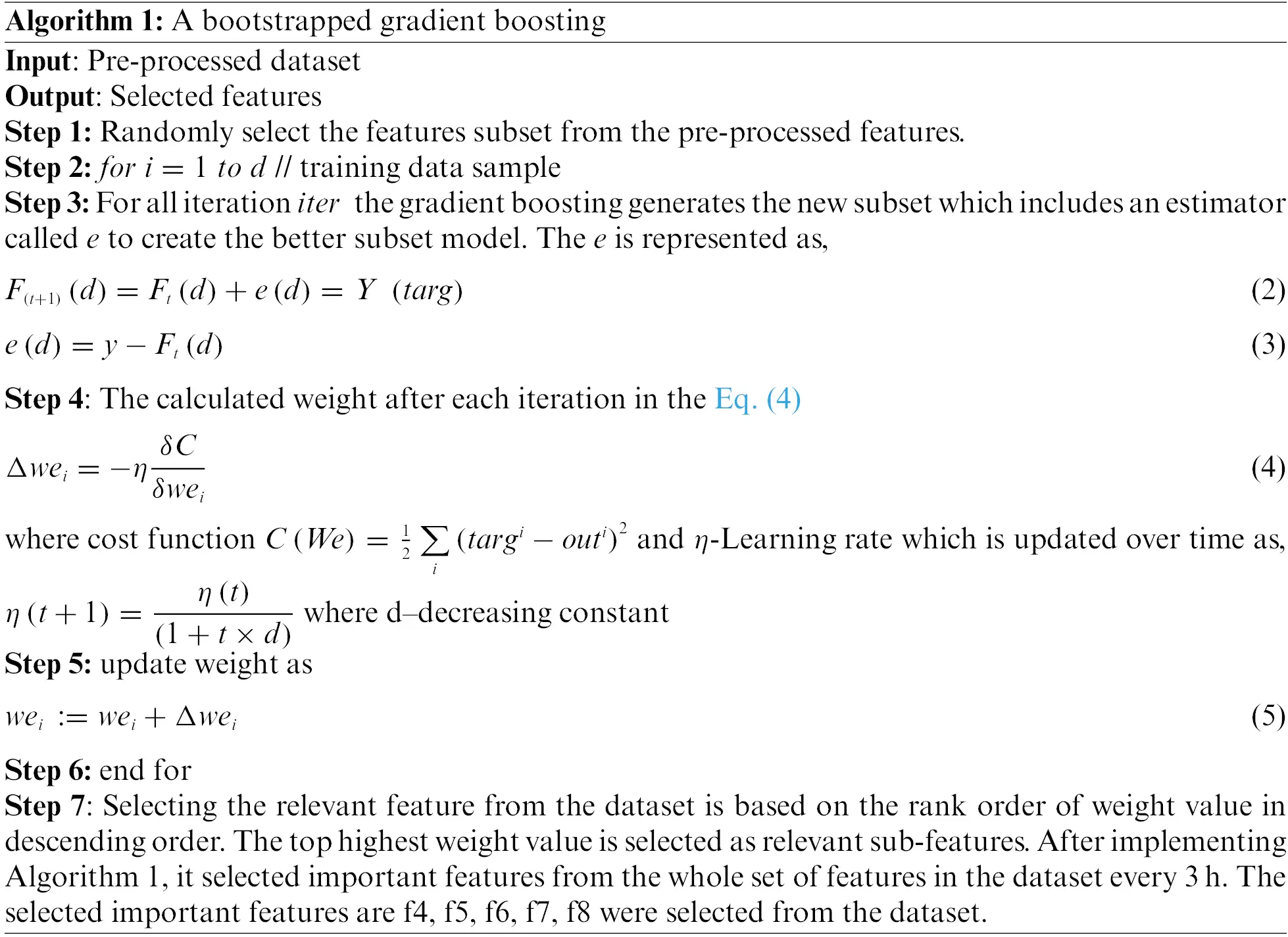3.4 Ensemble Learning Method

Ensemble methods are a combination of various algorithmic models. In our proposed model, we combine the results of Random Forest (RF) , Gradients Boosting Decision Tree (GBDT), Naïve Bayes Bernoulli (NBB), and KNN Algorithmic model. The final prediction outcome uses the outcome of the above algorithms which are then ensemble by max voting to produce a better result.

3.4.1 Random Forest

The random forest is a decision tree ensemble method in which weather data samples are classified based on many sub-trees. A classification outcome is generated for each tree which are then ensemble. Random Forest is similar to decision trees,

t(x,θn) for n=1,2N(6)

where θn-is an independent and identical distribution of random samples. Each prediction class K trees is given to a data sample D. The construction of the subset of decision tree is based on the following formula,

D={(xi,yi)} (|X|=n,xiRm, yiR(7)

The prediction probability of each subset is as follows,

prob(pc|F)=pc1+pc2++cpni=1n(pci(pc|F))(8)

where pc-is the prediction class, F-are the features, pc1,   pc2,., and pcn is the probability of each feature in the dataset, n is the number of subsets, and the error is calculated as,

errcorr(1s2)s2(9)

Here corr is the correlation between the trees and s  is the parametric strength of the tree. The random forest classifier is shown in Fig. 4. In the training dataset, n  is the subset of the decision tree which is partitioned and a prediction is calculated for every tree. In Fig. 3 the prediction of each tree is shown in different colour. As a whole, the average of all subsets of the trees’ prediction result is considered as the final prediction class of the random forest.Figure 4: Random forest

3.4.2 Gradient Boosting Decision Tree (GBDT)

This approach works like machine learning techniques and effective in the prediction of weather forecasting. It gathers large volume of weak models and changes the modules’ weight sample in every single step. GBDT is an ensemble boosting technique that iteratively generates decision trees based on its new regression value. This newly generated regression decision tree is used to fit the error in the classification of weather input dataset in every step of the process. The purpose of using GBDT is, it works with non-linear complex variable. It performs better in the training stage than the testing stage because it has no overfitting problems. The probability is calculated by logarithm of ratio between known feature attribute values to the unknown feature attribute data set. it can be defined as:

Pn(di)=n=1Nγndtn(di)(10)

Here Pn(di) is the probability of attributes in the weather forecasting model and it is evaluated from the N. regression trees dtn(di) and γn is the step length. At each iteration of the process, a weak decision tree  dt(di) is selected to reduce the loss function loss [yi,Pn(di)]. here yi is a value of observing di. Now the equation is formed as,

Pn(di)=Pn1dtn(di)+γndtn(di)(11)

Pn(di)=Pn1dtn(di)+argmini=1nloss[yi,Pn1(di)dtn(di)](12)

The gradient loss function can be defined as residual error between yi and Pn1. dtn(di) is expressed as:

Pn(di)=Pn1(d)+γni=1nloss[yi,Pn(di)](13)

In all iteration, a new weak module is created with respect to the last module-based errors. The GBDT module trains the weather data and produces a better prediction with low noise of the data.

3.4.3 Naive Bayes Bernoulli (NBB)

To improve the accurate analysis of weather forecasting Naïve Bayes technique is implemented. This technique is based on the concept of occurrence probability. And also, it shows the accurate outcome using attribute of the weather dataset and it receives the primitive process. The Bayes theorem is used in the NBB model and is defined as shown below:

prob(X|Y)=Prob(Y|X)prob(X)Prob(Y)(14)

In Eq. (14), the probability of Y is the evidence and X is the hypothesis. Here X and Y are the events. For finding the probability of X’s occasion, the Y’s occasion is a valid one. Then Y’s occasion is termed as proof. The probability of X is priori of X. Similarly, the probability of prob(X|Y) is deduced by Y.

3.4.4 KNN

KNN algorithm can be used for both predictive problems and classification of weather forecasting. Its analysis the weather input vector values of prediction values and observation values to generate the new set of data points. In the prediction of the weather condition [20,21], it uses a series of input data with different nearest neighbour values. In the weather attribute missing attribute, values are evaluated based on the similarity of attributes by using distance function. This paper uses Euclidean distance of each data from weather input data vector by using the following equation:

Dist(p,q)=i=1n(piqi)2(15)

Here P={p1, p2,,pn} and Q={q1 , q2 ,., qn}. The purpose of using KNN algorithm is predicting both numerical and categorical attribute values of weather dataset, missing values can be easily identified and also correlated data also considered. Notice that it is time consuming in the analysis of Big-data process.

3.5 Ensemble Learning for the Prediction of Weather Forecasting (EBWF)

An Ensemble Learning technique is a meta-algorithm that combines several ML algorithmic results into one model of prediction in order to improve the prediction rate. In this research, ensemble learning is implemented for prediction of the weather forecasting based on Random Forest, Gradient Boosting Decision Tree, Naive Bayes Bernoulli, and KNN Algorithms. The prediction outcomes of this evolutionary algorithm are then ensemble using max voting to obtain the best final result. This bagging concept is implemented by the following algorithm:

f(x)=1mi=1mfi(x)(16)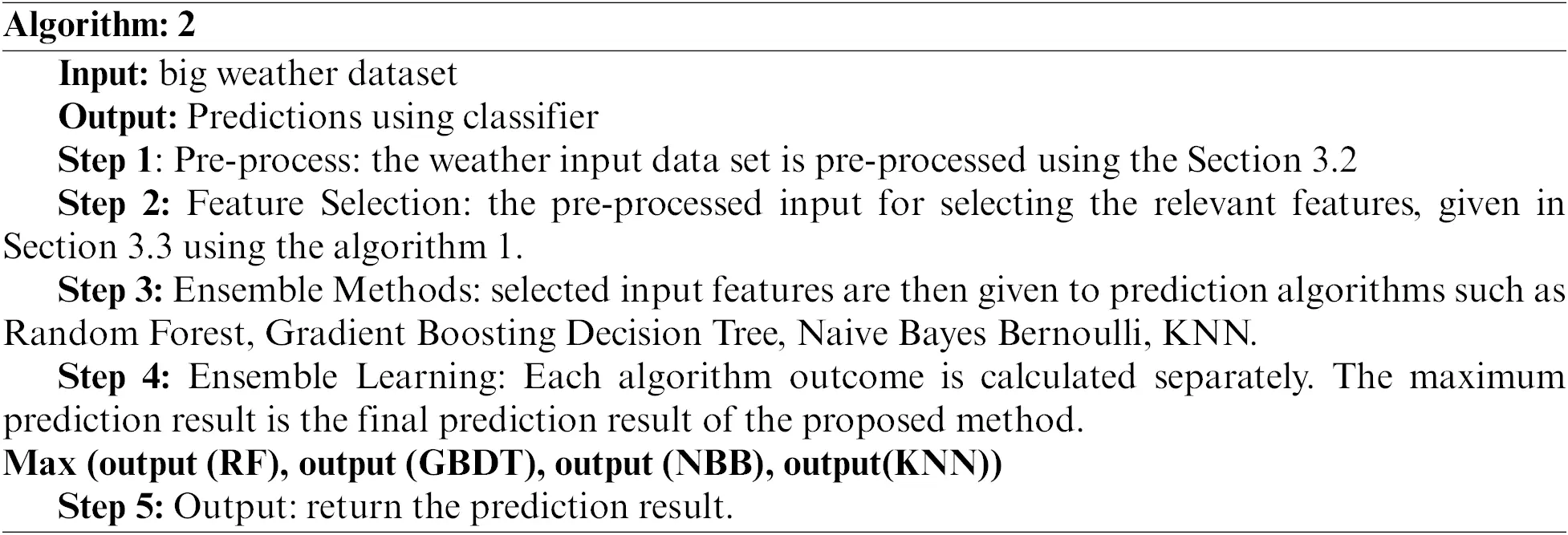4  Result and Discussion

4.1 Performance of Metric Measures for Performance Analysis

The proposed work provides the ensemble-based prediction of weather forecasting model using Random Forest, Gradient Boosting Decision Tree, Naive Bayes Bernoulli, and KNN Algorithm. For this we are using the following parametric metric measures:

Correlation Coefficient (CC)

It reflects the correlation between the attribute of weather forecasting and observation of attributes.

Corre=cov(A,Obs)Var(A)Var(Obs)(17)

Here, cov(A,Obs) is the covariance outcome of weather forecasting model.

Var(A) and Var(Obs) is the variance of the weather forecast model and observation of attributes in the weather forecasting model.

cov(A,Obs)=i=1n(AiA¯)(ObsiObs¯)(18)

Var(A)=i=1n(AiA¯)2(19)

Var(Obs)=i=1n(ObsiObs¯)2(20)

Classification Error Rate or Misclassification (CER)

It is used to calculate as the fraction of predictions were incorrect.

CER=(1Accuracy)(21)

Agreement Index

This is a measure of the prediction error in the weather forecasting model and it is calculated bas shown in the following formula:

IndexA=1i=1n(AiObsi)2i=1n(|Aiobs¯|+|ObsiObs¯|)2 0IndexA1(22)

The range of index agreement (IndexA) varies between 0 and 1; when the IndexA value is close to 1, that refers to an exact matching outcome and 0 refers no agreement at all.

Nash-Sutcliffe Efficiency Coefficient (NSE)

It is used to access the prediction of weather forecasting model based on numerical values and it can be calculated by:

NSE=1i=1n(AiObsi)2i=1n(ObsiObs¯)2(23)

NSE ranges from to 1. If the outcome of NSE is 1, that means it matches the observed attribute perfectly.

Prediction Time (PT)

It calculates the time required to predict the weather forecasting and it is calculated by using:

PT=ntime (EBWF)(24)

Here, time (EBWF) is the time taken for the prediction of weather forecasting algorithms like Random Forest, Gradient Boosting Decision Tree, Naive Bayes Bernoulli, and KNN Algorithms. time is evaluated in terms of ms. And n is the total number of big weather data in the dataset.

Multi-Class Confusion Matrix is used to represent the performance of a multi-class classification model in terms of True Positives (TP), True Negatives (TN), False Positives (FP), and False Negatives (FN).

Classification Accuracy

Classification accuracy=TP+TNTP+TN+FP+FNX100(25)

It is used to calculate as the ratio between number of correct classifications to the total number of classifications.

Classification Precision

Classification precision=TPTP+FPX100(26)

It is the ratio between correctly positive labelled classifier to the total number of all positive labels.

Classification Recall

Classification recall=TPTP+FN×100(27)

F1=2precisionRecallPrecision+Recall(28)

Specificity=TNTN+FP(29)

Tab. 3 show the comparison of Correlation coefficient (CC), Index Agreement (IA), and Nash-Sutcliffe Efficiency Coefficient (NSE).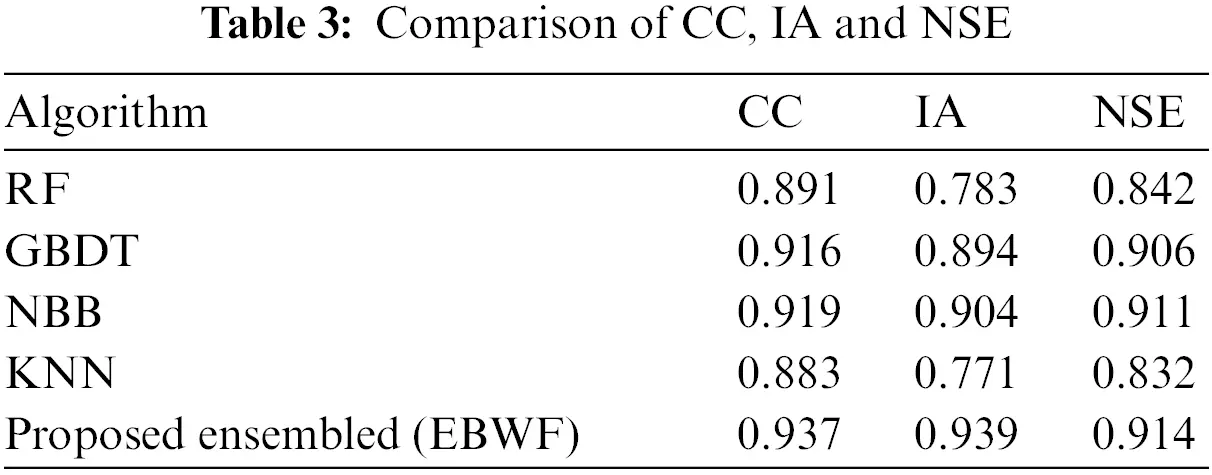Tab. 3 shows that the proposed ensemble produced the better result compared with other existing algorithms. Fig. 5 show the calculation of classification error rate using Eq. (21).

Fig. 5 shows that the proposed ensemble-based algorithm produces minimum error rate of 0.043 in the CER train data. The classification error rate of train data set is random forest got 0.116, gradient boost decision tree 0.074, NBB 0.061 and KNN got 0.107. In the test data, ensemble-based algorithm produces minimum error rate of 0.051. The classification error rate of test data set is random forest got 0.121, gradient boost decision tree 0.082, NBB 0.066 and KNN got 0.109. Fig. 6 show the prediction time of various ML algorithms.Figure 5: Classification error rate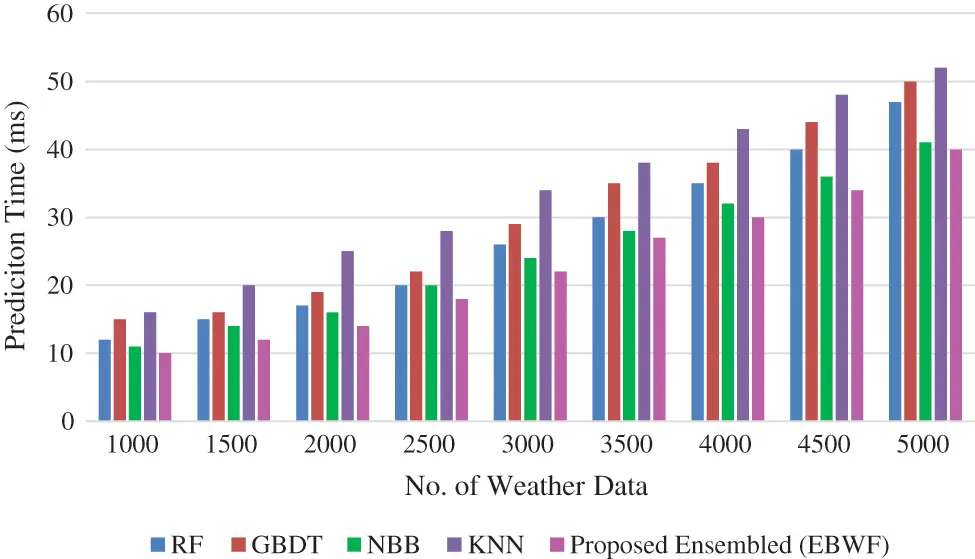Figure 6: Prediction time

Fig. 6 shows that the prediction time of the bigdata of weather input is analysed. There are various sizes of the data in the weather dataset. Our proposed EBWF technique requires less time to predict the weather forecasting. Tab. 4 shows the accuracy rate of both training (80%) and testing (20%) data in the weather dataset.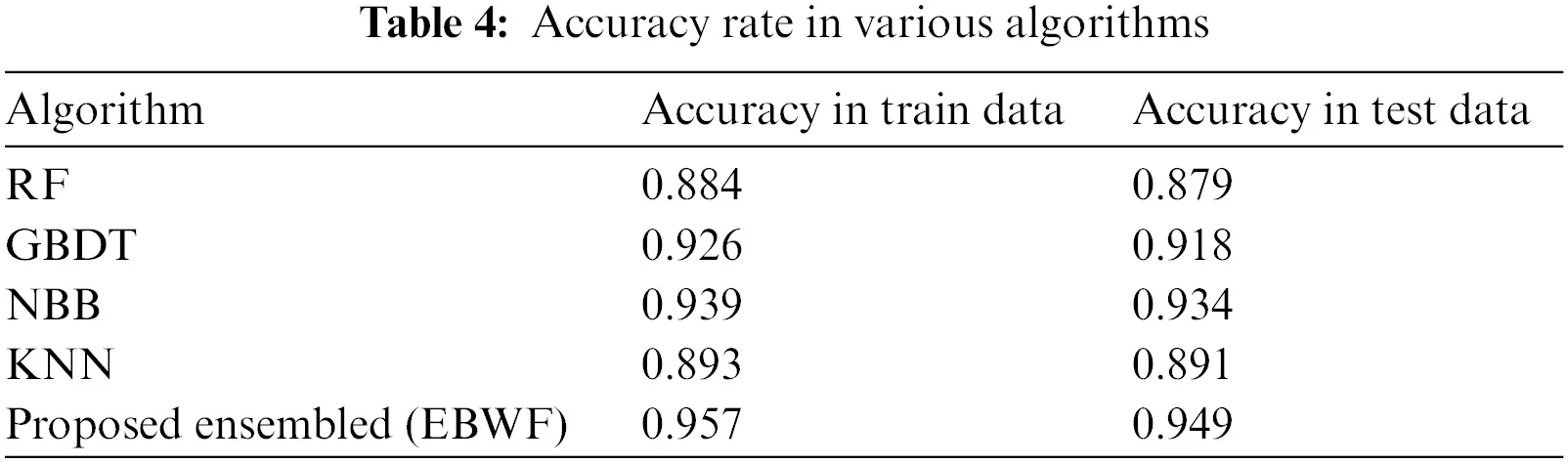In the Tab. 4, the results of various algorithms are compared based on Accuracy parametric measures in both training and testing dataset. The proposed ensembled based (EBWF) got 0.957 accuracy rate in the training data set and similarly for the testing dataset 0.949 accuracy rate. It gives better accuracy rate when comparing it with various algorithms. Tab. 5 shows the precision and recall rate of various algorithms.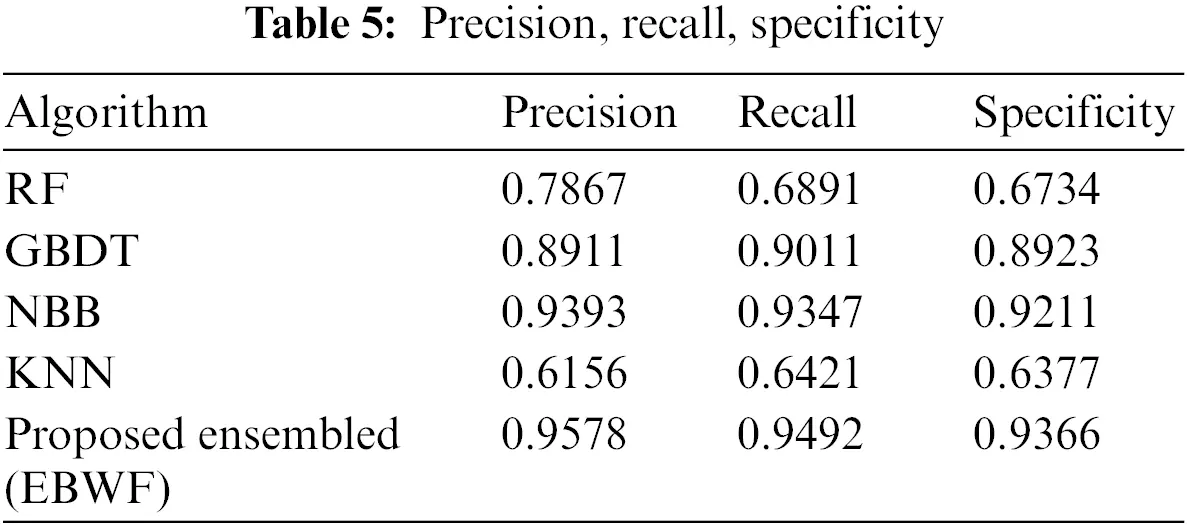In Tab. 5, the results of various algorithms are compared based on precision and recall parametric measures. The proposed ensembled based (EBWF) model got 0.9681 precision, 0.9592 recall, and 0.9366 specificity. Fig. 7 shows the F1-score metric rate of various algorithms.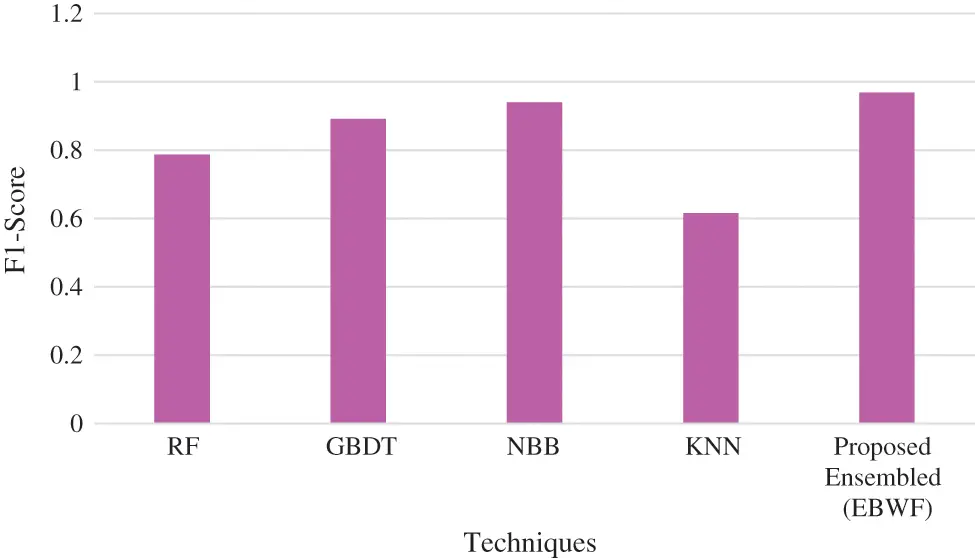Figure 7: F1-Score

Fig. 7 shows the comparison of F1-Score with various algorithms. The results show that our proposed work outperforms other methods. The performance of the proposed ensemble model with the proposed EBWF framework shows is better in terms of high accuracy, less error, and less prediction time. In this research, ensemble learning is implemented for prediction of the weather forecasting based on Random Forest, Gradient Boosting Decision Tree, Naive Bayes Bernoulli, and KNN Algorithms. Each algorithm outcome is calculated separately.

Max (output (RF), output (GBDT), output (NBB), output(KNN))

The prediction outcomes of this evolutionary algorithm are then ensemble using max voting to obtain the best final result. Fig. 8 shows that classifier performance of confusion matrix for the test data set with classes of {Cloudy, Rainy, Sun shine, Sun rise} with factors of confusion terms are TP, TN, FP, FN.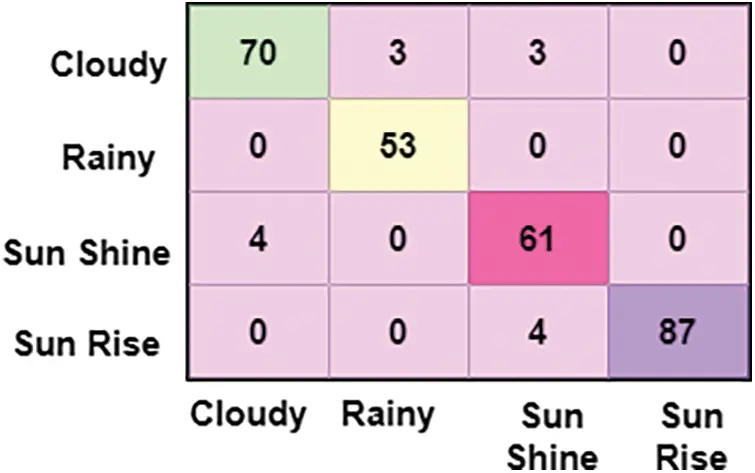Figure 8: Confusion matrix of test data PWFM

In the Fig. 8 describes that confusion matrix of testing data in the data set with the classifiers of cloudy, rainy, sun shine and sun rise for the ensemble based proposed work.

5  Conclusion

In this proposed work, ensemble model is compared with existing machine learning algorithms for predicting weather using essential performance measures. We have observed that ensemble based EBWF prediction system gives the best weather prediction results. The aim of this proposed work is to have high accuracy rate, low error, and less prediction time. For the evaluation purpose, we used airport weather data gathered from different stations in India. This research helps various communities like fishing, transport, farming etc. In fishing it alert population regarding weather condition. In farming it helps to plan plantations and irrigation. The results of the proposed EBWF model outperform other techniques which are Random Forest, KNN, GBDT, and NBB. The ensemble-based EBWF model resulted with 0.957 accuracy rates using the training data set and similarly 0.949 accuracy rate for the testing dataset. In our future work, research will explore by connecting IoT devices for collecting weather data which can help produce high accuracy in less perdition time and improve the decision-making process.

Acknowledgement: The authors would like to thank the Deanship of Scientific Research at Umm Al-Qura University for supporting this work by Grant Code: (22UQU4310373DSR10).

Funding Statement: The authors extend their appreciation to the Deanship of Scientific Research at King Khalid University for funding this work under grant number (RGP 2/42/43). Princess Nourah bint Abdulrahman University Researchers Supporting Project number (PNURSP2022R135), Princess Nourah bint Abdulrahman University, Riyadh, Saudi Arabia.

Conflicts of Interest: The authors declare that they have no conflicts of interest to report regarding the present study.

## References

1. H. Abdel Kader, M. Abd El Salam and M. Mohamed, “Hybrid machine learning model for rainfall forecasting,” Journal of Intelligent Systems and Internet of Things, vol. 1, no. 1, pp. 5–12, 129–156, 202
2. C. Z. Basha, N. Bhavana, P. Bhavya and V. Sowmya, “Rainfall prediction using machine learning and deep learning techniques,” in Proc. Int. Conf. on Electronics and Sustainable Communication Systems, Coimbatore, India, pp. 92–97, 2020.
3. A. H. M. Jakaria, M. D. Mosharaf Hossain and M. Ashiqur Rahman, “Smart weather forecasting using machine learning: A case study in Tennessee,” arXiv:2008.10789v1, 2020.
4. J. Cifuentes, G. Marulanda, A. Bello and J. Reneses, “Air temperature forecasting using machine learning techniques: A review,” Energies, vol. 13, no. 16, 2020.
5. S. Rasp, P. D. Dueben, S. Scher, J. A. Weyn, S. Mouatadid et al., “Weatherbench: A benchmark data set for data-driven weather forecasting,” Journal of Advances in Modeling Earth Systems, vol. 12, no. 11, 2020.
6. P. Hewage, M. Trovati, E. Pereira and A. Behera, “Deep learning based effective finegrained weather forecasting model,” Pattern Analysis and Applications, vol. 24, no. 1, pp. 343–366, 2021.
7. B. Wang, J. Lu, Z. Yan, H. Luo, T. Li et al., “Deep uncertainty quantification: A machine learning approach for weather forecasting,” in Proc. 25th ACM SIGKDD Int. Conf. on Knowledge Discovery & Data Mining, NewYork, USA, pp. 2087–2095, 2019.
8.    C. Z. Basha, N. Bhavana, P. Bhavya and V. Sowmya, “Rainfall prediction using machine learning and deep learning techniques,” in Int. Conf. on Electronics and Sustainable Communication Systems ICESC, Coimbatore, India, pp. 92–97, 2020.
9.    Z. Q. Huang, Y. C. Chen and C. Y. Wen, “Realtime weather monitoring and prediction using city buses and machine learning,” Sensors, vol. 20, no. 18, pp. 1–21, 2020.
10.  M. Mohammed, R. Kolapalli, N. Golla and S. S. Maturi, “Prediction of rainfall using machine learning techniques,” International Journal of Scientific and Technology Research, vol. 9, no. 01, pp. 3236–3240, 2020.
11.  A. M. Suresha, “Machine learning for mining weather patterns and weather forecasting,” Weather, x19149956, 2020.
12.  M. H. Yen, D. W. Liu, Y. C. Hsin, C. E. Lin and C. C. Chen, “Application of the deep learning for the prediction of rainfall in southern Taiwan,” Scientific Reports, vol. 9, no. 1, pp. 1–9, 2019.
13.  N. Singh, S. Chaturvedi and S. Akhter, “Weather forecasting using machine learning algorithm,” in Proc. Int. Conf. on Signal Processing and Communication (ICSC), Noida, India, pp. 171–174, 2019.
14.  A. Parashar, “IoT based automated weather report generation and prediction using machine learning,” in Proc. 2nd Int. Conf. on Intelligent Communication and Computational Techniques (ICCT), Jaipur, India, pp. 339–344, 2019.
15.  A. Mahabub and A. B. Habib, “An overview of weather forecasting for Bangladesh using machine learning techniques,” Machine Learning, pp. 1–36, 2019.
16. M. Sattar Hanoon, A. Najah Ahmed, N. Zaini, A. Razzaq, P. Kumar et al., “Developing machine learning algorithms for meteorological temperature and humidity forecasting,” Terengganu State in Malaysia, 2021.
17. L. Ma, G. Zhang and E. Lu, “Using the gradient boosting decision tree to improve the delineation of hourly rain areas during the summer from advanced Himawari imager data,” Journal of Hydrometeorology, vol. 19, no. 5, pp. 761–776, 2018.
18. M. G. Schultz, C. Betancourt, B. Gong, F. Kleinert, M. Langguth et al., “Can deep learning beat numerical weather prediction?,” Philosophical Transactions of the Royal Society A, vol. 379, pp. 20200097, 2021.
19. A. Marwa Farouk, A. Somia, M. Asklany and H. E. Wahab, “Data mining algorithms for weather forecast phenomena: Comparative study,” IJCSNS International Journal of Computer Science and Network Security, vol. 19, no. 9, 20
20. W. Chen, T. Sun, F. Bi, T. Sun, C. Tang et al., “Overview of digital image restoration,” Journal of New Media, vol. 1, no. 1, pp. 35–44, 2019.
21. X. R. Zhang, J. Zhou, W. Sun and S. K. Jha, “A lightweight CNN based on transfer learning for COVID-19 diagnosis,” Computers, Materials & Continua, vol. 72, no. 1, pp. 1123–1137, 2022.This work is licensed under a Creative Commons Attribution 4.0 International License, which permits unrestricted use, distribution, and reproduction in any medium, provided the original work is properly cited.